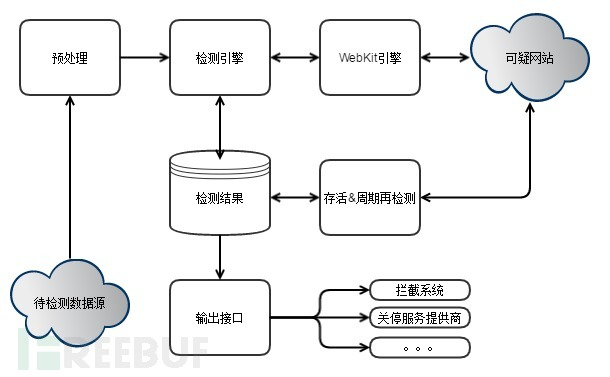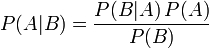FreeBuf+小程序2016-05-21 21:04:29

## 0x01基本系统架构## 0x02检测引擎

### 贝叶斯算法简介• P(A)是A的先验概率或边缘概率。之所以称为"先验"是因为它不考虑任何B方面的因素；
• P(A|B)是已知B发生后A的条件概率，也由于得自B的取值而被称作A的后验概率；
• P(B|A)是已知A发生后B的条件概率，也由于得自A的取值而被称作B的后验概率；
• P(B)是B的先验概率或边缘概率，也作标准化常量。

### 分类原理

• P(h+|W)=P(h+)*P(W|h+)/P(W)
• P(h-|W)=P(h-)*P(W|h-)/P(W)

P(W)为常量，可以暂时忽略。P(h+)、P(h-)为先验概率，即一个真实的网页集合中，钓鱼网页的比例与正常网页的比例。

P(wi|h+)经过Laplacean平滑处理后，P(wi|h+)=(1 + 特征词wi在h+训练集中的词频)  /  (全部特征词去重个数  +  h+下所有词出现总数)。

### 数据准备

Phantomjs：

``````var webPage = require('webpage');
var system = require('system');
var page = webPage.create();

if (system.args.length === 1) {
console.log("error");
phantom.exit();
} else {
url = system.args;
page.open(url, function (status) {
if (status == 'success') {
var content = page.content;
console.log(content);
} else {
console.log("error");
}
phantom.exit();
});
};
``````

Python：

``````def get_page_content(url):
cmd = 'phantomjs getPageContent.js %s' % (url)
stdout, stderr = subprocess.Popen(cmd, shell = True,
stdout = subprocess.PIPE, stderr = subprocess.PIPE).communicate()
return stdout
``````

### 建立模型

``````def get_chinese_content(raw_page_content):
content_list = re.findall(ur"[\u4e00-\u9fa5]+", raw_page_content)
return "".join(content_list)
``````

``````def get_seg_list(chinese_content):
return jieba.cut(chinese_content)
``````

``````一,一下,一个,一些,的,了,和,是,就,都,而,及,與,著,或,一何,一切......
``````

``````def count_all_unique_word(word_list):
return len(set(word_list))

all_unique_word_count = count_all_unique_word(all_word_list)
``````

``````def get_word_frequency(word_list):
frequency_dict = dict()
for w in word_list:
if frequency_dict.has_key(w):
frequency_dict[w] = frequency_dict[w] + 1
else:
frequency_dict[w] = 1
return frequency_dict

phish_word_frenquency_dict = get_word_frequency(phish_word_list)
normal_word_frequency_dict = get_word_frequency(normal_word_list)
``````

``````def get_word_probability_table(word_frequency_dict, category_word_count, all_unique_word_count):
probability_dict = dict()
for key, value in word_frequency_dict.items():
probability_dict[key] = (1 + value) / (all_unique_word_count + category_word_count)
return probability_dict

phish_word_probability_table = get_word_probability_table(phish_word_frequency_dict, all_phish_word_count, all_unique_word_count)
normal_word_probability_table = get_word_probability_table(normal_word_frequency_dict, all_normal_word_count, all_unique_word_count)
``````

P(h+)、P(h-)我们可以直接求出，即训练样本集中钓鱼网页数目与正常网页数目的比例probability_phish，probability_normal。

### 钓鱼检测

``````def analyse_page(raw_page_content, probability_phish, probability_normal, phish_word_probability_table, normal_word_probability_table):
page_word_list = get_seg_list(get_chinese_content(raw_page_content))
p_phish = probability_phish
p_normal = probability_normal
for w in page_word_list:
p_phish = p_phish * phish_word_probability_table[w]
p_normal = p_phish * normal_word_probability_table[w]
return True if p_phish > p_normal else False
``````

## 0x03 策略、改进

“钓鱼攻击”者为了躲避反钓鱼系统的检测，常常采取屏蔽反钓鱼检测系统IP，屏蔽特定UA，页面使用js或flash进行动态渲染，全页面图片化等手段。

### *本文作者：阿里云誉反欺诈（企业帐号），转载须注明来自FreeBuf黑客与极客（FreeBuf.COM）

# 钓鱼 # 检测 # 系统

+ 收入我的专栏• 0 文章数
• 0 评论数
• 0 关注者